• ## 基数

2020-12-28 19:20:56
基数指一种进制中组成的基本数字，也就是不能再进行拆分的数字，例如十进制是0~9，二进制是0和1，八进制是0-7，十六进制则是0-9，A-F（大小写均可）。 也可以这样进行记忆，假设是n进制的话,，基数就是[0，n-1]范围...
基数指一种进制中组成的基本数字，也就是不能再进行拆分的数字，例如十进制是0~9，二进制是0和1，八进制是0-7，十六进制则是0-9，A-F（大小写均可）。 也可以这样进行记忆，假设是n进制的话,，基数就是[0，n-1]范围的数字，基数的个数和进制值相等，如：十进制有10个基数，以此类推…
展开全文• 基数排序： 思想：基数排序可以看成是桶排序的扩展，以整数排序为例，主要思想是将整数按位数划分，准备 10 个桶，代表 0 – 9，根据整数个位数字的数值将元素放入对应的桶中，之后按照输入赋值到原序列中，依次对十...
• /// 基数排序 /// </summary> public class Program { public static void Main(string[] args) { int[] array = { 43, 69, 11, 72, 28, 21, 56, 80, 48, 94, 32, 8 }; RadixSort(array, 10); ShowSord...shell
• convesion_gui 是一个小的 MATLAB GUI，用于将基数 (2-36) 中的任何数字转换为另一个基数 (2-36)。 它还支持浮点数。matlab
• 排序算法1.1 算法的之间的比较1.2 冒泡排序1.3 选择排序1.4 插入排序1.5 快速排序1.6 堆排序1.7 计数排序1.8 桶排序1.9 基数排序1.10 希尔排序1.11 归并排序 1. 排序算法 1.1 算法的之间的比较 排序算法 平均...


文章目录
1. 排序算法1.1 算法的之间的比较1.2 冒泡排序1.3 选择排序1.4 插入排序1.5 快速排序1.6 堆排序1.7 计数排序1.8 桶排序1.9 基数排序1.10 希尔排序1.11 归并排序

1. 排序算法
1.1 算法的之间的比较
排序算法平均时间复杂度最差时间复杂度空间复杂度数据对象稳定性冒泡排序O(n2)O(n2)O(1)稳定选择排序O(n2)O(n2)O(1)数组不稳定、链表稳定插入排序O(n2)O(n2)O(1)稳定快速排序O(n*log2n)O(n2)O(log2n)不稳定堆排序O(n*log2n)O(n*log2n)O(1)不稳定归并排序O(n*log2n)O(n*log2n)O(n)稳定希尔排序O(n*log2n)O(n2)O(1)不稳定计数排序O(n+m)O(n+m)O(n+m)稳定桶排序O(n)O(n)O(m)稳定基数排序O(k*n)O(n2)O(k+n)稳定
1.2 冒泡排序
算法思想
比较相邻的元素，如果第一个比第二个大，就交换他们两个。对每一对相邻元素作同样的工作，从第一对开始，一直到最后一对，做完后，最后的元素会是最大的元素。针对所有的元素重复上面的步骤，除排序好的。持续对越来越少的元素重复上述步骤，直到哪次没有任何一对数字需要比较或者是交换。
图解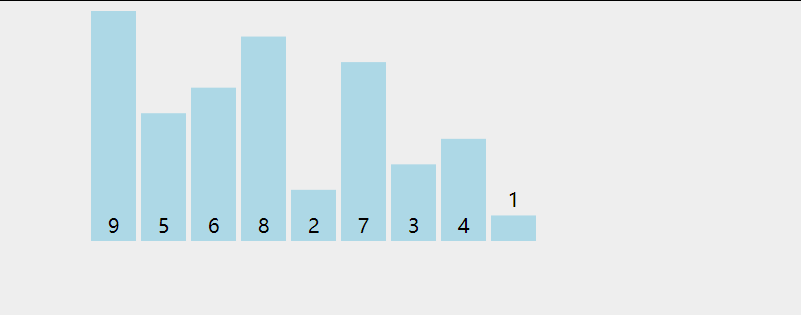代码
void bubbleSort(int a[], int n)
{
for (int i = 0; i < n - 1; i++)
{
for (int j = 0; j < n - i - 1; ++j)
{
if (a[j] > a[j + 1])
{
swap(a[j], a[j + 1]);
}
}
}
}

1.3 选择排序
算法思想
在未排序的序列中找到最小（大）元素，存放到排序序列的起始位置。从剩下未排序元素中继续寻找最小（大）元素，然后放到自己已排序的序列的末尾。以此类推，直到所有元素排序完毕。
图解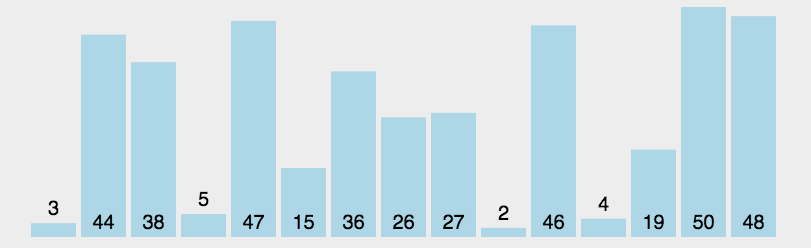代码
void selectionSort(int a[], int len)
{
int min;
for (int i = 0; i < len - 1; i++)
{
min = i;
for (int j = i + 1; j < len; j++)
{
if (a[j] < a[min])
{
min = j;
}
}
swap(a[i], a[min]);
}
}

1.4 插入排序
算法思想
从第一个元素开始，该元素可以认为已经被排序。取出下一个元素，在已经排序的元素序列中从后向前扫描。如果该元素（已排序）大于新元素，该元素移到下一个位置。重复步骤3，直到找到已排序的元素小于或者等于新的元素的位置。将元素插入到对应位置。重复2~5。
图解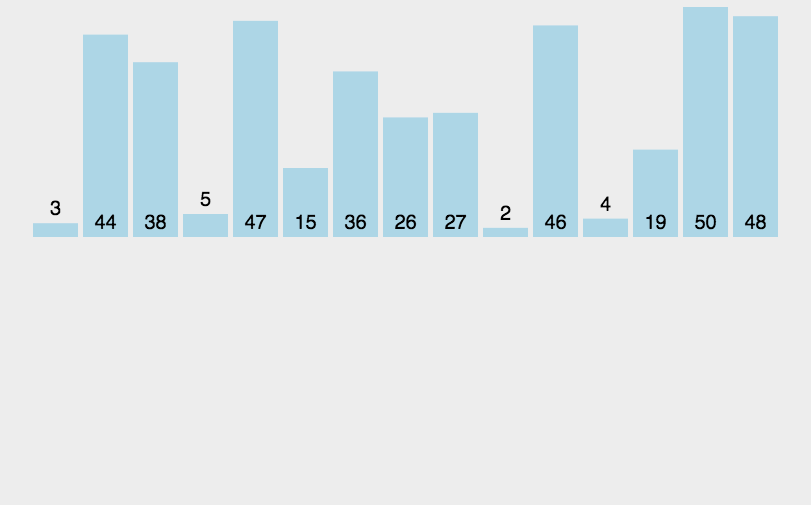代码
void InsertSort(int a[], int n)
{
for (int i = 1; i < n; i++)
{
if (a[i] < a[i - 1])
{
int val = a[i];
int j = i - 1;
a[j + 1] = a[j];
while (j > 0 && val < a[j - 1])
{
a[j] = a[j - 1];
j--;
}
a[j] = val;
}
}
}

1.5 快速排序
算法思想
选第一个数为标准。将比基准小的数据交换到前面，比基准大的交换到后面对左边的空间和右边的空间重复，直到各区间只有一个数字
图解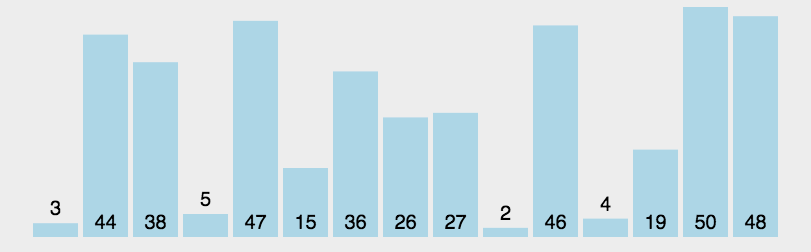代码
void QuickSort(int a[], int left, int right)
{
if (left >= right)
return;
int begin = left;
int end = right;
//keyi定在右边，需要从左边开始找
int keyi = end;
int key = a[keyi];

while (begin < end)
{
//因为我们从小往大排
//从左边开始找 找大
while (begin < end && a[begin] <= key)
begin++;
a[end] = a[begin];

//从右边开始找 找小
while (begin < end && a[end] >= key)
end--;
a[begin] = a[end];
}
a[end] = key;

QuickSort(a, left, end - 1);
QuickSort(a, end + 1, right);
}

1.6 堆排序
算法思想
如果要从小到大排序，建立大堆，根节点大于左右子树。将根结和最后一个元素交换，并且树的元素个数减1。重复1~2，直到只剩一个元素。
图解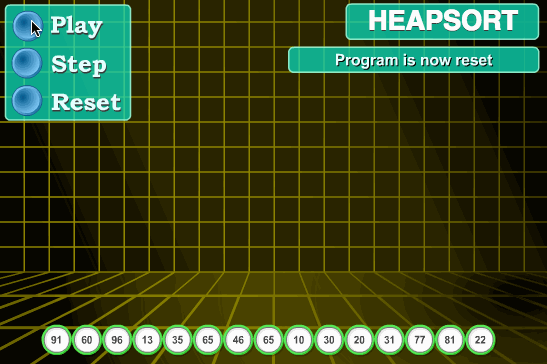代码
void AdjustDown(int* a, int n, int root)//n是大小，root是根节点的下标
{
int parent = root;
int child = parent * 2 + 1;

while (child < n)
{
//child指向左右孩子中最小的那个
if (child + 1 < n && a[child + 1] < a[child])
{
child++;
}
if (a[child] < a[parent])
{
swap(a[child], a[parent]);
parent = child;
child = parent * 2 + 1;
}
else
{
break;
}
}
}

void HeapPop(Heap* php)
{
assert(php);
assert(php->size > 0);

swap(&php->a, &php->a[php->size - 1]);
php->size--;

}

1.7 计数排序
算法思想
找出待排序的数组最大和最小的元素统计数组中每个值为i的元素出现的个数，存入数组c的第i-min项将下标+min的值根据在数组c中的个数存到原数组中。
图解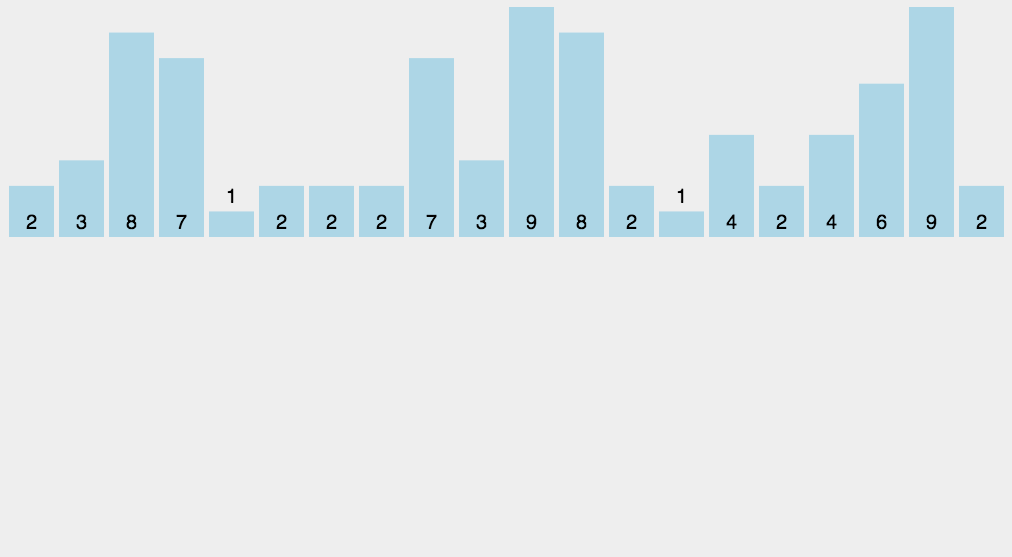代码
void CountSort(int a[], int len)
{
int max = a;
int min = a;
for (int i = 0; i < len; i++)
{
if (a[i] > max)
max = a[i];
if (a[i] < min)
min = a[i];
}
int l = max - min;//计算数组最大最小值的差
int* count_a = new int[l + 1];
for (int i = 0; i < l + 1; i++)
{
count_a[i] = 0;
}

for (int i = 0; i < len; i++)
{
count_a[a[i] - min]++;//统计元素个数
}

int j = 0;
for (int i = 0; i < len; i)
{
while (j <= l && count_a[j])
{
count_a[j]--;
a[i] = j + min;
i++;
}
j++;
}
}

1.8 桶排序
算法思想
设置一个定量的数组当作空桶；遍历输入数据，并且把数据一个一个放到对应的桶里去；对每个不是空的桶进行排序；从不是空的桶里把排好序的数据拼接起来。
图解1.9 基数排序
算法思想
图解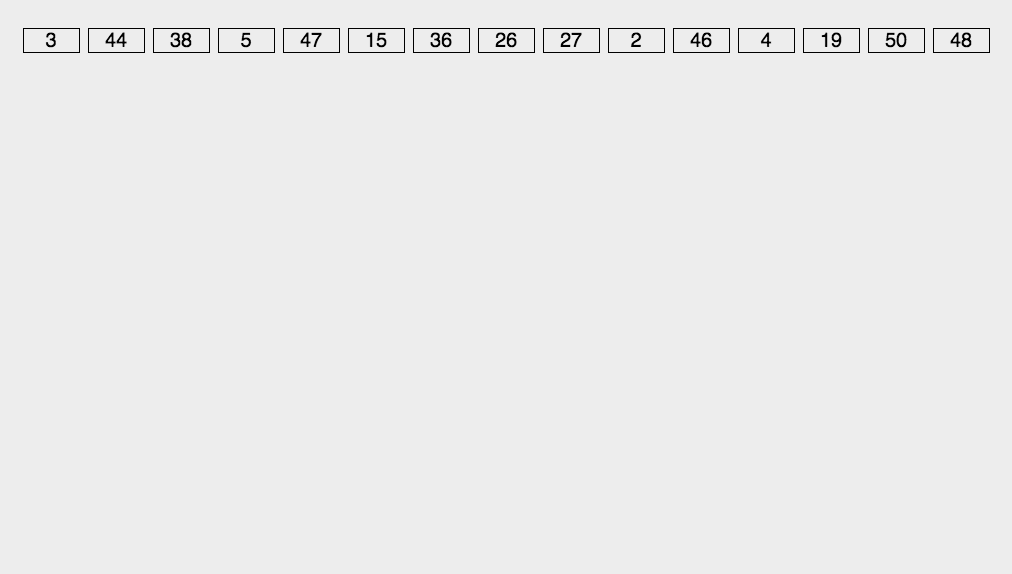1.10 希尔排序
算法思想
选择一个增量序列t1,t2,…,tk,其中ti>tj,tk = 1。按照增量序列个数k，对序列进行k趟排序每趟排序根据对应的增量ti，将待排序的序列分割成若干长度为m的子序列，分别对各子表进行直接插入排序。
图解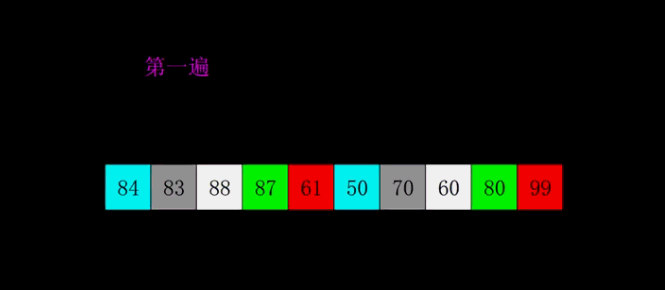代码
void ShellSort(int* a, int n)
{
printf("原数组->", gap);
PrintArray(a, n);

int gap = n;//间隔
while (gap > 1)
{
gap = gap / 3 + 1;//保证最后一趟一定为1
for (int i = 0; i < n - gap; i++)
{
int end = 0;
int tmp = a[end + gap];
while (end >= 0)
{
if (tmp < a[end])
{
a[end + gap] = a[end];
end -= gap;
}
else
{
break;
}
}

a[end + gap] = tmp;
}
printf("gap:%d->", gap);
PrintArray(a, n);
}
}

1.11 归并排序
算法思想
把长度为n的输入序列分成两个长度为n/2的子序列；对这两个子序列分别采用归并排序；将两个排序好的子序列合并成一个最终的排序序列。
图解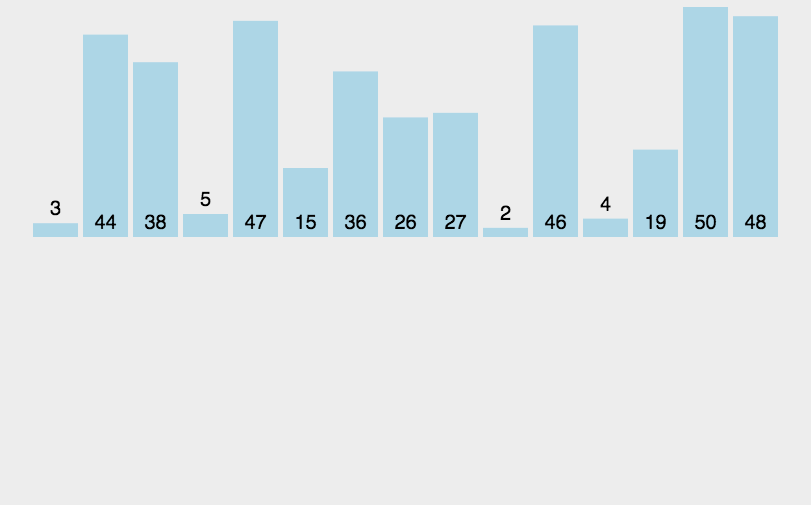代码
void _MergeSort(int* a, int left, int right, int* tmp)
{
if (left == right)
return;

//int mid = (left + right) >> 1;//有溢出风险
int mid = left + ((right - left) >> 1);

_MergeSort(a, left, mid, tmp);
_MergeSort(a, mid + 1, right, tmp);

int begin1 = left, end1 = mid;
int begin2 = mid + 1, end2 = right;
int i = begin1;

while (begin1 <= end1 && begin2 <= end2)
{
if (a[begin1] < a[begin2])
{
tmp[i++] = a[begin1++];
}
else
{
tmp[i++] = a[begin2++];
}
}

while (begin1 <= end1)
{
tmp[i++] = a[begin1++];

}
while (begin2 <= end2)
{
tmp[i++] = a[begin2++];
}

//拷回原数组
int j = left;
while (j <= right)
{
a[j] = tmp[j];
j++;
}
}

void MergeSort(int* a, int n)
{
int* tmp = (int*)malloc(sizeof(int)*n);
_MergeSort(a, 0, n - 1, tmp);
free(tmp);
}

展开全文• ## 排序算法系列：基数排序

万次阅读 多人点赞 2016-06-16 23:14:07
这也是基数排序的魅力所在，基数排序可以理解成是建立在“计数排序”的基础之上的一种排序算法。在实际项目中，如果对效率有所要求，而不太关心空间的使用时，我会选择用计数排序（当然还有一些其他的条件），或是...
引言
今天要说的这个排序算法很特殊，它不需要直接对元素进行相互比较，也不需要将元素相互交换，你需要做的就是对元素进行“分类”。这也是基数排序的魅力所在，基数排序可以理解成是建立在“计数排序”的基础之上的一种排序算法。在实际项目中，如果对效率有所要求，而不太关心空间的使用时，我会选择用计数排序（当然还有一些其他的条件），或是一些计数排序的变形。

版权说明
著作权归作者所有。 商业转载请联系作者获得授权，非商业转载请注明出处。 本文作者：Q-WHai 发表日期： 2016年6月16日 本文链接：https://qwhai.blog.csdn.net/article/details/51695211 来源：CSDN 更多内容：分类 >> 算法与数学

基数排序
数据背景
在基数排序中，我们不能再只用一位数的序列来列举示例了。一位数的序列对基数排序来说就是一个计数排序。 这里我们列举无序序列 T = [ 2314, 5428, 373, 2222, 17 ]
排序原理
上面说到基数排序不需要进行元素的比较与交换。如果你有一些算法的功底，或者丰富的项目经验，我想你可能已经想到了这可能类似于一些“打表”或是哈希的做法。而计数排序则是打表或是哈希思想最简单的实现。
计数排序
计数排序的核心思想是，构建一个足够大的数组 hashArray[]，数组大小需要保证能够把所有元素都包含在这个数组上 。 假设我们有无序序列 T = [ 2314, 5428, 373, 2222, 17 ] 首先初始化数组 hashArray[] 为一个全零数组。当然，在 Java 里，这一步就不需要了，因为默认就是零了。 在对序列 T 进行排序时，只要依次读取序列 T 中的元素，并修改数组 hashArray[] 中把元素值对应位置上的值即可。这一句有一些绕口。打个比方，我们要把 T 映射到 hashArray[] 中，就是 hashArray[T] = 1. 也就是 hashArray = 1. 如果序列 T 中有两个相同元素，那么在 hashArray 的相应位置上的值就是 2。 下图是计数排序的原理图： （假设有无序序列：[ 5, 8, 9, 1, 4, 2, 9, 3, 7, 1, 8, 6, 2, 3, 4, 0, 8 ]）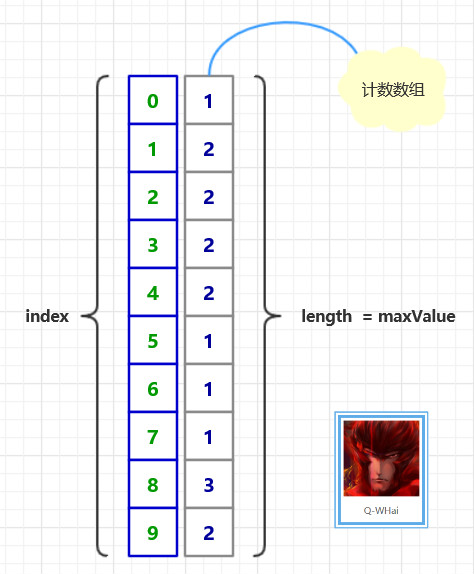基数排序原理图
上面的计数排序只是一个引导，好让你可以循序渐进地了解基数排序。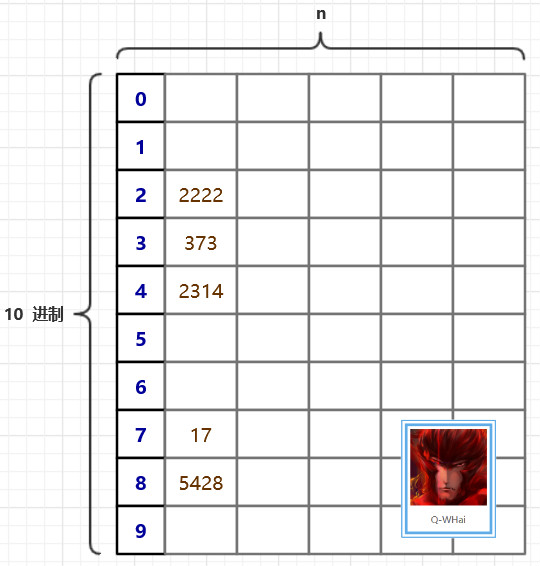上面这幅图，或许你已经在其他的博客里见到过。这是一个很好的引导跟说明。在基数排序里，我们需要一个很大的二维数组，二维数组的大小是 （10 * n）。10 代表的是我们每个元素的每一位都有 10 种可能，也就是 10 进制数。在上图中，我们是以每个数的个位来代表这个数，于是，5428 就被填充到了第 8 个桶中了。下次再进行填充的时候，就是以十位进行填充，比如 5428 在此时，就会选择以 2 来代表它。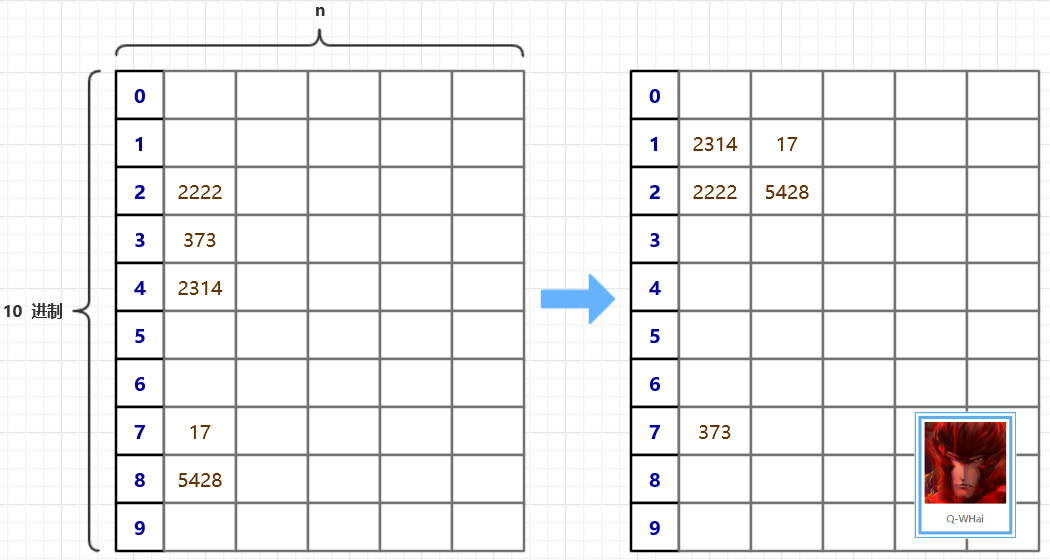算法优化
在算法的原理中，我们是以一张二维数组的表来存储这些无序的元素。使用二维数组有一个很明显的不足就是二维数组太过稀疏。数组的利用率为 10%。 在寻求优化的路上，我们想到一种可以压缩空间的方法，且时间复杂度并没有偏离得太厉害。那就是设计了两个辅助数组，一个是 count[]，一个是 bucket[]。count 用于记录在某个桶中的最后一个元素的下标，然后再把原数组中的元素计算一下它应该属于哪个“桶”，并修改相应位置的 count 值。直到最大数的最高位也被添加到桶中，或者说，当所有的元素都被被在第 0 个桶中，基数排序就结束了。 优化后的原理图如下：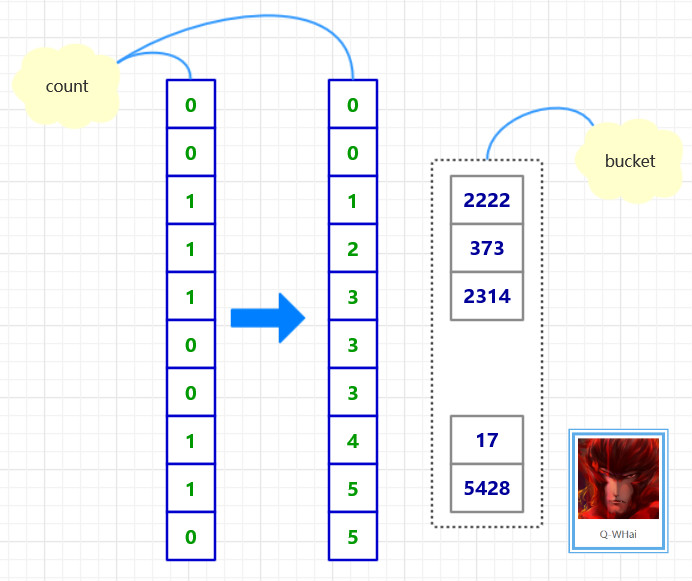算法实现
import org.algorithm.array.sort.interf.Sortable;

/**
* <p>
* 基数排序/桶排序
* </p>
* 2016年1月19日
*
* @author <a href="http://weibo.com/u/5131020927">Q-WHai</a>
* @see <a href="http://blog.csdn.net/lemon_tree12138">http://blog.csdn.net/lemon_tree12138</a>
* @version 0.1.1
*/
public class RadixSort implements Sortable {

@Override
public int[] sort(int[] array) {
if (array == null) {
return null;
}

int maxLength = maxLength(array);

return sortCore(array, 0, maxLength);
}

private int[] sortCore(int[] array, int digit, int maxLength) {
if (digit >= maxLength) {
return array;
}

final int radix = 10; // 基数
int arrayLength = array.length;
int[] bucket = new int[arrayLength];

// 统计将数组中的数字分配到桶中后，各个桶中的数字个数
for (int i = 0; i < arrayLength; i++) {
count[getDigit(array[i], digit)]++;
}

// 将各个桶中的数字个数，转化成各个桶中最后一个数字的下标索引
for (int i = 1; i < radix; i++) {
count[i] = count[i] + count[i - 1];
}

// 将原数组中的数字分配给辅助数组 bucket
for (int i = arrayLength - 1; i >= 0; i--) {
int number = array[i];
int d = getDigit(number, digit);
bucket[count[d] - 1] = number;
count[d]--;
}

return sortCore(bucket, digit + 1, maxLength);
}

/*
* 一个数组中最大数字的位数
*
* @param array
* @return
*/
private int maxLength(int[] array) {
int maxLength = 0;
int arrayLength = array.length;
for (int i = 0; i < arrayLength; i++) {
int currentLength = length(array[i]);
if (maxLength < currentLength) {
maxLength = currentLength;
}
}

return maxLength;
}

/*
* 计算一个数字共有多少位
*
* @param number
* @return
*/
private int length(int number) {
return String.valueOf(number).length();
}

/*
* 获取 x 这个数的 d 位数上的数字
* 比如获取 123 的 0 位数,结果返回 3
*
* @param x
* @param d
* @return
*/
private int getDigit(int x, int d) {
int a[] = { 1, 10, 100, 1000, 10000, 100000, 1000000, 10000000, 100000000, 1000000000 };
return ((x / a[d]) % 10);
}
}

基数排序过程图
如果我们的无序是 T = [ 2314, 5428, 373, 2222, 17 ]，那么其排序的过程就如下两幅所示。 基数排序过程图-1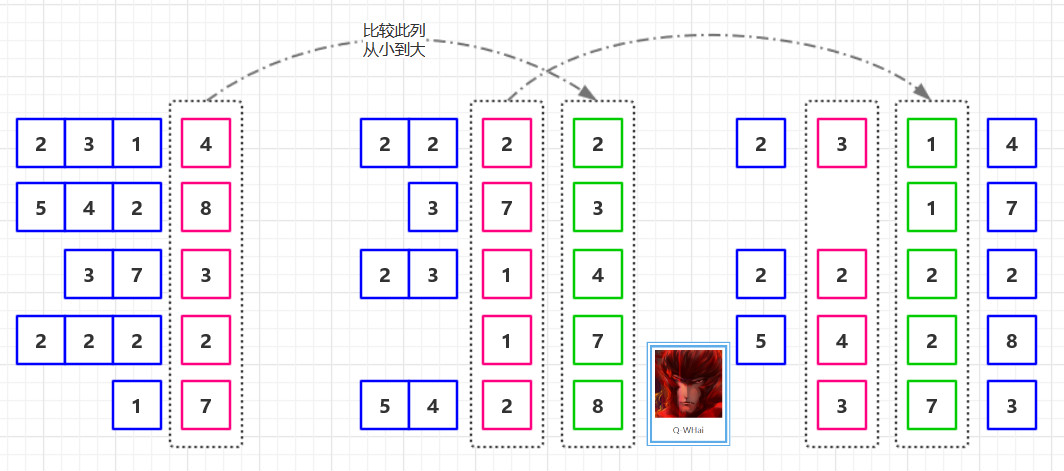基数排序过程图-2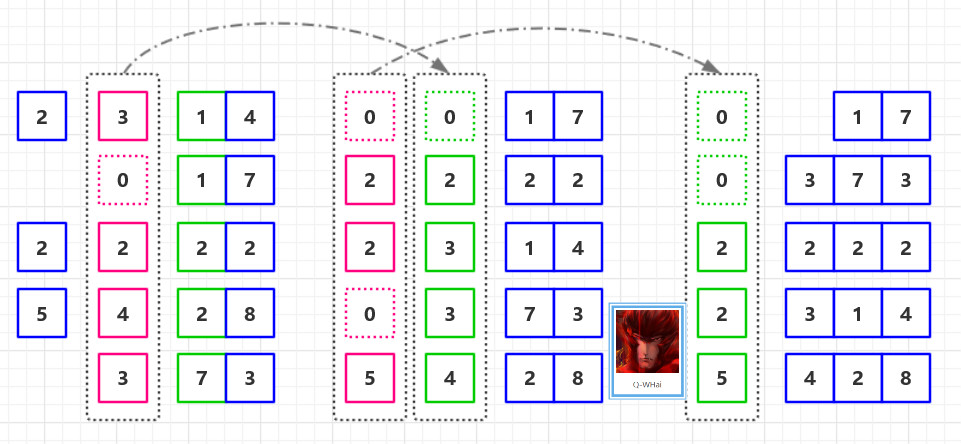复杂度分析
排序方法时间复杂度空间复杂度稳定性复杂性平均情况最坏情况最好情况基数排序O(d*(n+r))O(d*(n+r))O(d*(n+r))O(n+r)稳定较复杂 其中，d 为位数，r 为基数，n 为原数组个数。 在基数排序中，因为没有比较操作，所以在复杂上，最好的情况与最坏的情况在时间上是一致的，均为 O(d * (n + r))。

Ref
《算法导论》

https://github.com/qwhai/algorithms-sort

征集
如果你也需要使用ProcessOn这款在线绘图工具，可以使用如下邀请链接进行注册： https://www.processon.com/i/56205c2ee4b0f6ed10838a6d
展开全文排序算法 计数排序 桶排序
•Java
• 基数基数 2、基数 10、基数 16、基数 64 用于表示值的符号数。 编码：用于表示特定位置数字值的符号。 例子： Base 10 十进制到 Base 16 十六进制： cnvbase('76','0123456789','0123456789ABCDEF') = '4C' ...matlab
• 村庄基数转换小程序，蛀牙应用于三调地类和村庄规划地类转换
• 基数排序，有链式的，也有非链式的，想要下载，我都有。数据结构...